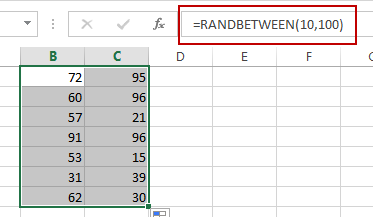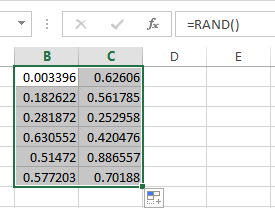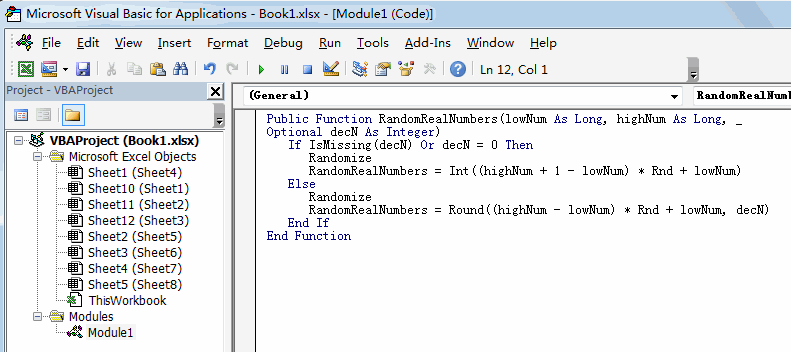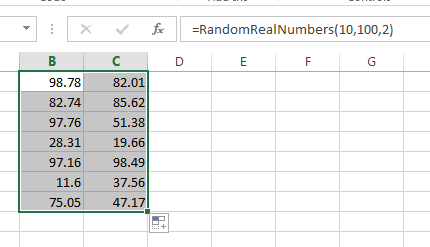# How to Generate Random Numbers between Two Numbers in Excel

This post will guide you how to generate random numbers between two numbers in Excel. How do I generate random numbers between two numbers without duplicates with VBA macro in Excel.

## Generate Random Numbers between Two Numbers with Formula

If you want to generate random numbers between two given numbers, you can use a formula based on the RANDBETWEEEN function in Excel.

For example, you need to generate random numbers between 10 and 100 numbers, you can use the following formula:

`=RANDBETWEEN(10,100)`

Type this formula into a blank cell, and press Enter key on your keyboard, and then drag the AutoFill handle to other cells to generate more random numbers.If you want to generate random number between 0 and 1, you can directly use the RAND function. Like this:

`=RAND()`## Generate Random Numbers between Two Numbers with VBA

You can also use VBA code to define a new function to achieve the same result of generating random numbers with 2 decimal places between two given numbers in Excel. Or you can generate random numbers with given decimal places in a given range of cells in Excel. Here are the steps:

#1 open your excel workbook and then click on “Visual Basic” command under DEVELOPER Tab, or just press “ALT+F11” shortcut.#2 then the “Visual Basic Editor” window will appear.

#3 click “Insert” ->”Module” to create a new module.#4 paste the below VBA code into the code window. Then clicking “Save” button.```Public Function RandomRealNumbers(lowNum As Long, highNum As Long, _
Optional decN As Integer)
If IsMissing(decN) Or decN = 0 Then
Randomize
RandomNumbers = Int((highNum + 1 - lowNum) * Rnd + lowNum)
Else
Randomize
RandomNumbers = Round((highNum - lowNum) * Rnd + lowNum, decN)
End If
End Function```

#5 back to the current worksheet, then type the following formula in a blank cell, and then press Enter key.

=RandomRealNumbers(10,100,2)

#6 drag the AutoFill handle over other cells to  generate random numbers with two decimal places between two given numbers 10 and 100.### Related Functions

• Excel RANDBETWEEN Function
The Excel RANDBETWEEN function returns a random integer number that is between the numbers you specify.The syntax of the RANDBETWEEN function is as below:= RANDBETWEEN (bottom,top)….

Sidebar